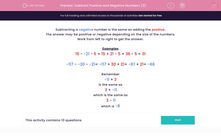# Subtract Positive and Negative Numbers (3)

In this worksheet, students subtract three positive and negative numbers.This content is premium and exclusive to EdPlace subscribers.Key stage:  KS 2

Curriculum topic:   Maths and Numerical Reasoning

Curriculum subtopic:   Mixed Problems

Difficulty level:#### Worksheet Overview

Subtracting a negative number is the same as adding the positive.

The answer may be positive or negative depending on the size of the numbers.

Work from left to right to get the answer.

Examples

15 - -21 - 5 = 15 + 21 - 5 = 36 - 5 = 31

-117 - -30 - -21= -117 + 30 + 21= -87 + 21= -66

Remember

-11 + 3

is the same as

3 + -11

which is the same as

3 - 11

which is -8

### What is EdPlace?

We're your National Curriculum aligned online education content provider helping each child succeed in English, maths and science from year 1 to GCSE. With an EdPlace account you’ll be able to track and measure progress, helping each child achieve their best. We build confidence and attainment by personalising each child’s learning at a level that suits them.

Get started